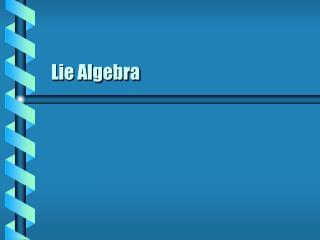DownloadDownload PresentationLie Algebra

# Lie Algebra

Download Presentation## Lie Algebra

- - - - - - - - - - - - - - - - - - - - - - - - - - - E N D - - - - - - - - - - - - - - - - - - - - - - - - - - -
##### Presentation Transcript

1. Lie Algebra

2. Lie Algebra • The set of all infinitessimal generators is the Lie algebra of the group G. • Linear transformation: • Let A be a matrix • G = {a; aI + eA;e << 1} • Product maps to a sum. S1 a  A b  B (I+eA)(I+eB) = I + e(A+B) + e2AB I + e(A+B) ab A+B

3. Group commutator: aba-1b-1 Use power series Discard high order terms Non-abelian group Lie bracket [A, B] = AB – BA Lie algebra includes addition, subtraction and bracket operations Lie Commutator S1 (I+eA)-1 = I – eA + e2A2– o(e3) (I+eA)(I+eB)(I+eA )-1 (I+eB )-1 = (I+e(A+B)+e2AB)(I–eA+e2A2)(I–eB+e2B2) I + e2(AB - BA)

4. Bracket Properties • Distributive • Antisymmetric • Jacobi identity S1 [A + B, C] =[A, C] + [B, C] [kA, B] = k[A, B] [A, B] = -[B, A] [A, [B, C]]+ [B, [C, A]]+ [C, [A, B]] = 0

5. Two vector fields acting on a scalar one-form. Obeys the three laws of a vector field. Obeys rules for a Lie bracket Vector Field Commutator

6. The Lie bracket between two vector fields. Measures the gap in an infinitessimal shift on a surface. Can be applied to functions and one-forms as well. Use the local coordinates of the vector field. Lie Derivative eh e2[x,h] ex ex p eh next